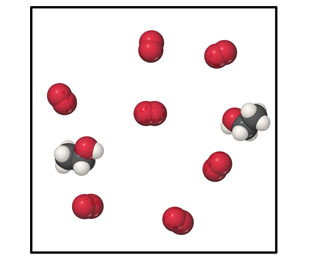# Problem: Consider the mixture of ethanol, C2H5OH, and O2 shown in the accompanying diagram.Write a balanced equation for the combustion reaction that occurs between ethanol and oxygen.

###### FREE Expert Solution

Combustion reaction: hydrocarbon reacts with Oxygen to form CO2 and H2O.

Complete reaction:  C2H5OH + O→ CO2 + H2O

• to balance, add coefficient so that the number of each atom for each type of element for both sides of the reaction are equal
96% (252 ratings)###### Problem Details

Consider the mixture of ethanol, C2H5OH, and O2 shown in the accompanying diagram.Write a balanced equation for the combustion reaction that occurs between ethanol and oxygen.

Frequently Asked Questions

What scientific concept do you need to know in order to solve this problem?

Our tutors have indicated that to solve this problem you will need to apply the Balancing Chemical Equations concept. You can view video lessons to learn Balancing Chemical Equations. Or if you need more Balancing Chemical Equations practice, you can also practice Balancing Chemical Equations practice problems.